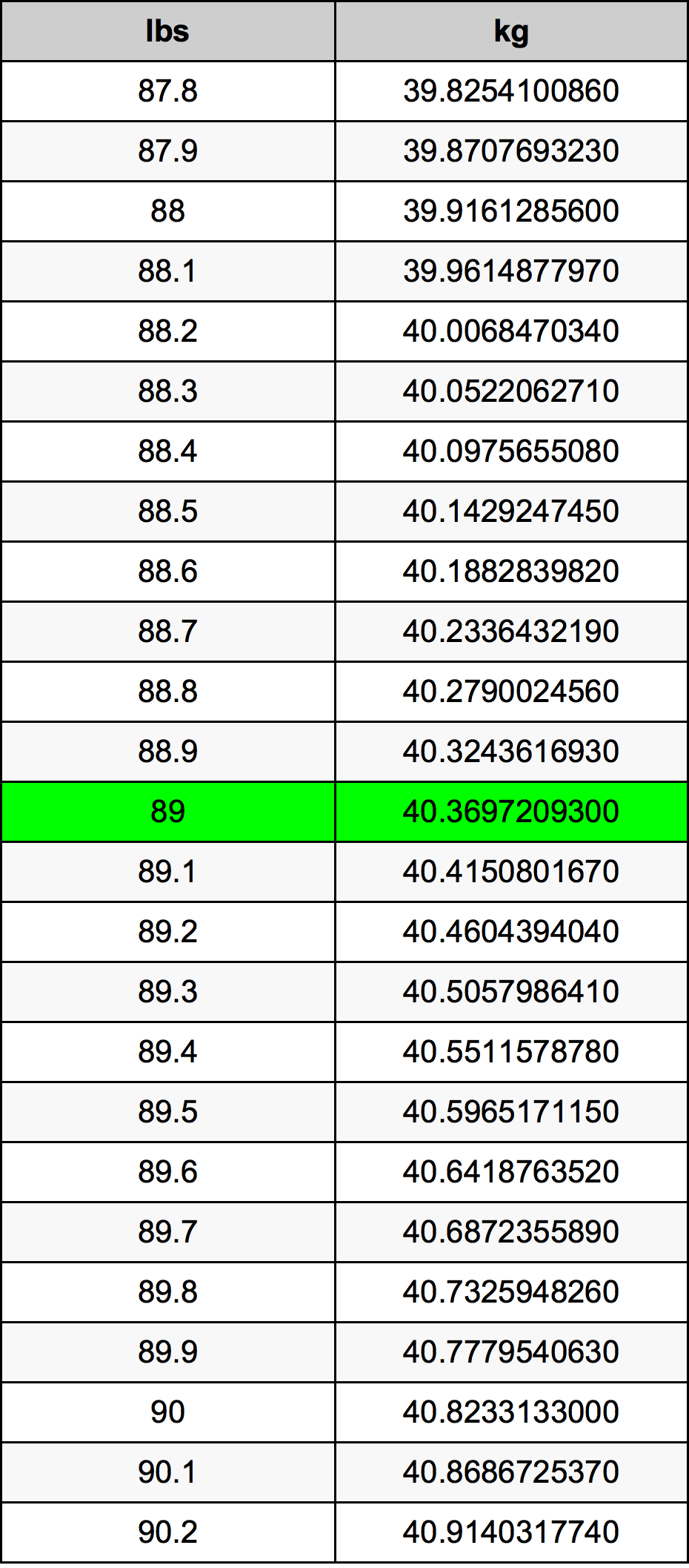Pounds To Kg

# 89 lbs to kg89 Pounds to Kilograms

lbs
=
kg

## How to convert 89 pounds to kilograms?

 89 lbs * 0.45359237 kg = 40.36972093 kg 1 lbs
A common question is How many pound in 89 kilogram? And the answer is 196.211413344 lbs in 89 kg. Likewise the question how many kilogram in 89 pound has the answer of 40.36972093 kg in 89 lbs.

## How much are 89 pounds in kilograms?

89 pounds equal 40.36972093 kilograms (89lbs = 40.36972093kg). Converting 89 lb to kg is easy. Simply use our calculator above, or apply the formula to change the length 89 lbs to kg.

## Convert 89 lbs to common mass

UnitMass
Microgram40369720930.0 µg
Milligram40369720.93 mg
Gram40369.72093 g
Ounce1424.0 oz
Pound89.0 lbs
Kilogram40.36972093 kg
Stone6.3571428571 st
US ton0.0445 ton
Tonne0.0403697209 t
Imperial ton0.0397321429 Long tons

## What is 89 pounds in kg?

To convert 89 lbs to kg multiply the mass in pounds by 0.45359237. The 89 lbs in kg formula is [kg] = 89 * 0.45359237. Thus, for 89 pounds in kilogram we get 40.36972093 kg.

## 89 Pound Conversion Table## Alternative spelling

89 Pounds to Kilograms, 89 Pounds in Kilograms, 89 lbs to kg, 89 lbs in kg, 89 lb to Kilogram, 89 lb in Kilogram, 89 lb to kg, 89 lb in kg, 89 lbs to Kilograms, 89 lbs in Kilograms, 89 Pound to Kilograms, 89 Pound in Kilograms, 89 Pound to kg, 89 Pound in kg, 89 Pounds to Kilogram, 89 Pounds in Kilogram, 89 lb to Kilograms, 89 lb in Kilograms